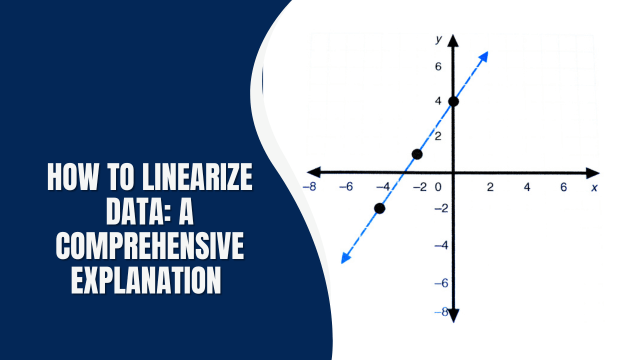# How to Linearize Data: A comprehensive ExplanationIn the realm of data analysis, the ability to linearize data is a crucial skill. Linearizing data means transforming non-linear relationships into linear ones, simplifying complex data sets and making them more amenable to analysis. Whether you’re a seasoned data scientist or a beginner looking to improve your data manipulation skills, this article will provide you with the knowledge and techniques you need to effectively linearize data.

### 1. Understanding Linearization

Linearization is the process of converting data that exhibits a non-linear relationship into a linear one. This transformation makes it easier to apply statistical and mathematical models, allowing for more accurate predictions and analysis.

### 2. Why Linearization Matters

Linearization is essential in various fields such as finance, engineering, and science. It simplifies complex data, making it easier to interpret and model. Linearized data often leads to more precise results in regression analysis, which is crucial for making informed decisions.

### 3. When to Linearize Data

Knowing when to linearize data is key. It’s usually necessary when relationships between variables in your data set appear curved or exponential. Identifying these instances can significantly improve the accuracy of your analysis.

### 4.1. Logarithmic Transformation

Logarithmic transformation is a common method for linearizing data. It involves taking the logarithm of one or more variables to create a linear relationship.

### 4.2. Exponential Transformation

Conversely, exponential transformation can be applied when data follows an exponential pattern. It involves exponentiating the data to achieve linearity.

### 4.3. Square Root Transformation

Square root transformation is useful for data with square relationships. It can help in making the data linear and improving model performance.

### 4.4. Box-Cox Transformation

The Box-Cox transformation is a versatile technique that can handle various types of non-linear data. It optimizes the transformation to achieve the best linear fit.

### 5. Steps to Linearize Data

To linearize data effectively, follow these steps:

1. Identify non-linear patterns in your data.
2. Select the appropriate transformation technique.
3. Apply the chosen transformation to your data set.
4. Validate the linearity of the transformed data.
5. Proceed with your analysis using the linearized data.

### 6.1. Over-Transforming Data

Applying too many transformations can lead to distorted results. It’s essential to strike a balance and avoid excessive manipulation.

### 6.2. Neglecting Data Assumptions

Ensure that the assumptions of your chosen transformation technique are met. Failing to do so can lead to inaccurate results.

### 7. Real-World Applications

Linearization finds applications in various fields, including:

• Finance: Analyzing financial data to predict market trends.
• Engineering: Evaluating the behavior of materials under stress.
• Medicine: Modeling the spread of diseases in a population.

How To Flush Topamax Out Of Your System: A Comprehensive Guide

### Can I linearize data without mathematical software?

Yes, you can perform linearization manually with tools like Microsoft Excel by applying the appropriate transformation functions.

### What if my data is highly non-linear?

In cases of extreme non-linearity, you may need to consider advanced techniques or consult with a data expert.

### Are there automated tools for linearization?

Yes, there are software packages like Python’s SciPy library that offer automated data transformation functions.

### Can linearized data still have errors?

Yes, linearization simplifies data but does not eliminate errors. Always validate your results and consider uncertainties.

### Is linearization always the best approach?

No, it depends on the specific data and the goals of your analysis. Sometimes, non-linear modeling is more appropriate.